$$\newcommand{\id}{\mathrm{id}}$$ $$\newcommand{\Span}{\mathrm{span}}$$ $$\newcommand{\kernel}{\mathrm{null}\,}$$ $$\newcommand{\range}{\mathrm{range}\,}$$ $$\newcommand{\RealPart}{\mathrm{Re}}$$ $$\newcommand{\ImaginaryPart}{\mathrm{Im}}$$ $$\newcommand{\Argument}{\mathrm{Arg}}$$ $$\newcommand{\norm}{\| #1 \|}$$ $$\newcommand{\inner}{\langle #1, #2 \rangle}$$ $$\newcommand{\Span}{\mathrm{span}}$$

# 4.1E:

$$\newcommand{\vecs}{\overset { \rightharpoonup} {\mathbf{#1}} }$$

$$\newcommand{\vecd}{\overset{-\!-\!\rightharpoonup}{\vphantom{a}\smash {#1}}}$$

## Parametric Equations

For the following exercises, sketch the curves below by eliminating the parameter t. Give the orientation of the curve.

1) $$\displaystyle x=t^2+2t, y=t+1$$

Solution: orientation: bottom to top2) $$\displaystyle x=cos(t),y=sin(t),(0,2π]$$

3) $$\displaystyle x=2t+4,y=t−1$$

Solution: orientation: left to right4) $$\displaystyle x=3−t,y=2t−3,1.5≤t≤3$$

For the following exercises, eliminate the parameter and sketch the graphs.

5) $$\displaystyle x=2t^2,y=t^4+1$$

Solution: $$\displaystyle y=\frac{x^2}{4}+1$$For the following exercises, use technology (CAS or calculator) to sketch the parametric equations.

6) [T] $$\displaystyle x=t^2+t,y=t^2−1$$

7) [T] $$\displaystyle x=e^{−t},y=e^{2t}−1$$

Solution: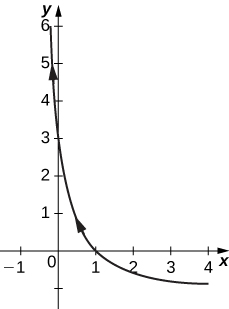8) [T] $$\displaystyle x=3cost,y=4sint$$

9) [T] $$\displaystyle x=sect,y=cost$$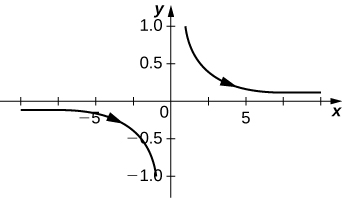For the following exercises, sketch the parametric equations by eliminating the parameter. Indicate any asymptotes of the graph.

10) $$\displaystyle x=e^t,y=e^{2t}+1$$

11) $$\displaystyle x=6sin(2θ),y=4cos(2θ)$$

Solution:12) $$\displaystyle x=cosθ,y=2sin(2θ)$$

13) $$\displaystyle x=3−2cosθ,y=−5+3sinθ$$

Solution: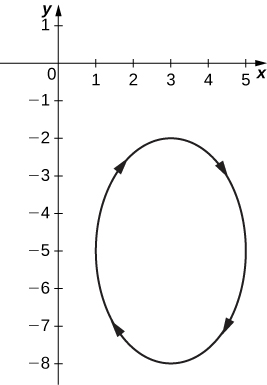14) $$\displaystyle x=4+2cosθ,y=−1+sinθ$$

15) $$\displaystyle x=sect,y=tant$$

Solution: Asymptotes are $$\displaystyle y=x$$ and $$\displaystyle y=−x$$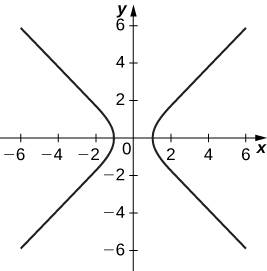16) $$\displaystyle x=ln(2t),y=t^2$$

17) $$\displaystyle x=e^t,y=e^{2t}$$

Solution:18) $$\displaystyle x=e^{−2t},y=e^{3t}$$

19) $$\displaystyle x=t^3,y=3lnt$$

Solution:20) $$\displaystyle x=4secθ,y=3tanθ$$

For the following exercises, convert the parametric equations of a curve into rectangular form. No sketch is necessary. State the domain of the rectangular form.

21) $$\displaystyle x=t^2−1,y=\frac{t}{2}$$

Solution: $$\displaystyle x=4y^2−1;$$ domain: $$\displaystyle x∈[1,∞)$$.

22) $$\displaystyle x=\frac{1}{\sqrt{t+1}},y=\frac{t}{1+t},t>−1$$

23) $$\displaystyle x=4cosθ,y=3sinθ,t∈(0,2π]$$

Solution: $$\displaystyle \frac{x^2}{16}+\frac{y^2}{9}=1;$$ domain $$\displaystyle x∈[−4,4].$$

24) $$\displaystyle x=cosht,y=sinht$$

25) $$\displaystyle x=2t−3,y=6t−7$$

Solution: $$\displaystyle y=3x+2;$$ domain: all real numbers.

26) $$\displaystyle x=t^2,y=t^3$$

27) $$\displaystyle x=1+cost,y=3−sint$$

Solution: $$\displaystyle (x−1)^2+(y−3)^2=1$$; domain: $$\displaystyle x∈[0,2]$$.

28) $$\displaystyle x=\sqrt{t},y=2t+4$$

29) $$\displaystyle x=sect,y=tant,π≤t<\frac{3π}{2}$$

Solution: $$\displaystyle y=\sqrt{x^2−1}$$; domain: $$\displaystyle x∈[−1,1]$$.

30) $$\displaystyle x=2cosht,y=4sinht$$

31) $$\displaystyle x=cos(2t),y=sint$$

Solution: $$\displaystyle y^2=\frac{1−x}{2};$$ domain: $$\displaystyle x∈[2,∞)∪(−∞,−2].$$

32) $$\displaystyle x=4t+3,y=16t^2−9$$

33) $$\displaystyle x=t^2,y=2lnt,t≥1$$

Solution: $$\displaystyle y=lnx;$$ domain: $$\displaystyle x∈(0,∞).$$

34) $$\displaystyle x=t3^,y=3lnt,t≥1$$

35) $$\displaystyle x=t^n,y=nlnt,t≥1,$$ where n is a natural number

Solution: $$\displaystyle y=lnx;$$ domain: $$\displaystyle x∈(0,∞).$$

36) $$\displaystyle x=ln(5t)$$ $$\displaystyle y=ln(t^2)$$ where $$\displaystyle 1≤t≤e$$

37) $$\displaystyle x=2sin(8t)$$ $$\displaystyle y=2cos(8t)$$

Solution: $$\displaystyle x^2+y^2=4;$$ domain: $$\displaystyle x∈[−2,2].$$

38) $$\displaystyle x=tant$$ $$\displaystyle y=sec^2t−1$$

For the following exercises, the pairs of parametric equations represent lines, parabolas, circles, ellipses, or hyperbolas. Name the type of basic curve that each pair of equations represents.

39) $$\displaystyle x=3t+4$$ $$\displaystyle y=5t−2$$

Solutin: line

40) $$\displaystyle x−4=5t$$ $$\displaystyle y+2=t$$

41) $$\displaystyle x=2t+1$$ $$\displaystyle y=t^2−3$$

Solution: parabola

42) $$\displaystyle x=3cost$$ $$\displaystyle y=3sint$$

43) $$\displaystyle x=2cos(3t)$$ $$\displaystyle y=2sin(3t)$$

Solution: circle

44) $$\displaystyle x=cosht$$ $$\displaystyle y=sinht$$

45) $$\displaystyle x=3cost$$ $$\displaystyle y=4sint$$

Solutin: ellipse

46) $$\displaystyle x=2cos(3t)$$ $$\displaystyle y=5sin(3t)$$

47) $$\displaystyle x=3cosh(4t)$$ $$\displaystyle y=4sinh(4t)$$

Solution: hyperbola

48) $$\displaystyle x=2cosht$$ $$\displaystyle y=2sinht$$

49) Show that $$\displaystyle x=h+rcosθ$$ $$\displaystyle y=k+rsinθ$$ represents the equation of a circle.

50) Use the equations in the preceding problem to find a set of parametric equations for a circle whose radius is 5 and whose center is $$\displaystyle (−2,3)$$.

For the following exercises, use a graphing utility to graph the curve represented by the parametric equations and identify the curve from its equation.

51) [T] $$\displaystyle x=θ+sinθ$$ $$\displaystyle y=1−cosθ$$

Solution: The equations represent a cycloid.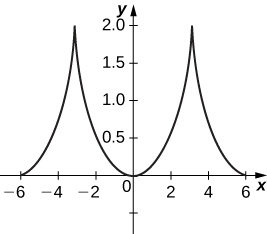52) [T] $$\displaystyle x=2t−2sint$$ $$\displaystyle y=2−2cost$$

53) [T] $$\displaystyle x=t−0.5sint$$ $$\displaystyle y=1−1.5cost$$

Solution:54) An airplane traveling horizontally at 100 m/s over flat ground at an elevation of 4000 meters must drop an emergency package on a target on the ground. The trajectory of the package is given by $$\displaystyle x=100t,y=−4.9t^2+4000,t≥0$$ where the origin is the point on the ground directly beneath the plane at the moment of release. How many horizontal meters before the target should the package be released in order to hit the target?

55) The trajectory of a bullet is given by $$\displaystyle x=v_0(cosα)ty=v_0(sinα)t−\frac{1}{2}gt^2$$ where $$\displaystyle v_0=500m/s, g=9.8=9.8m/s^2$$, and $$\displaystyle α=30degrees.$$ When will the bullet hit the ground? How far from the gun will the bullet hit the ground?

Solution: 22,092 meters at approximately 51 seconds.

56) [T] Use technology to sketch the curve represented by $$\displaystyle x=sin(4t),y=sin(3t),0≤t≤2π$$.

57) [T] Use technology to sketch $$\displaystyle x=2tan(t),y=3sec(t),−π<t<π.$$

Solution:58) Sketch the curve known as an epitrochoid, which gives the path of a point on a circle of radius b as it rolls on the outside of a circle of radius a. The equations are

$$\displaystyle x=(a+b)cost−c⋅cos[\frac{(a+b)t}{b}]$$ $$\displaystyle y=(a+b)sint−c⋅sin[\frac{(a+b)t}{b}]$$.

Let $$\displaystyle a=1,b=2,c=1. 59) [T] Use technology to sketch the spiral curve given by \(\displaystyle x=tcos(t),y=tsin(t)$$ from $$\displaystyle −2π≤t≤2π.$$60) [T] Use technology to graph the curve given by the parametric equations $$\displaystyle x=2cot(t),y=1−cos(2t),−π/2≤t≤π/2.$$ This curve is known as the witch of Agnesi.

61) [T] Sketch the curve given by parametric equations $$\displaystyle x=cosh(t)$$ $$\displaystyle y=sinh(t),$$ where $$\displaystyle −2≤t≤2.$$

Solution: